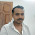6 Factors Affecting The Permeability - Civil Engineering

## 6 Factors Affecting The Permeability

Permeability of a soil sample depends upon the following 6 factors.
6 factors affecting the permeability are:

### 1) Size and shape of particles

The permeability varies approximately as the square of the grain size. The permeability of coarse grained soil is inversely proportional to the specific surface of particles at a given porosity.

### 2) Properties of the pore fluid

The permeability is directly proportional to the unit weight of water and inversely proportional to its viscosity.Again the viscosity change with the change in temperature. Normally ,with the increase in temperature viscosity decreases and hence permeability increases.

### 3) Void ratio of soil

The permeability (k) is directly proportional to c.e3/ (1+e), where 'c' is a factor and 'e' is the void ratio.Hence permeability decrease with decrease in void ratio & vice-versa.

### 4) Structural arrangement of soil particles

The permeability may vary with different geometric arrangement and shape of voids.

### 5) Entrapped air and organic impurities

The permeability is greatly reduced if air is entrapped in the voids.Presence of organic foreign matter in soil mass also decreases the permeability.

### 6) Adsorbed water

The adsorbed water surrounding the fine soil particles reduces the effective voids available for the passage of water and thereby reduces the permeability.

#### 1 comment:

1.